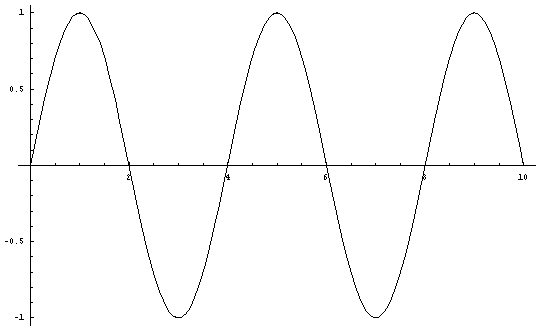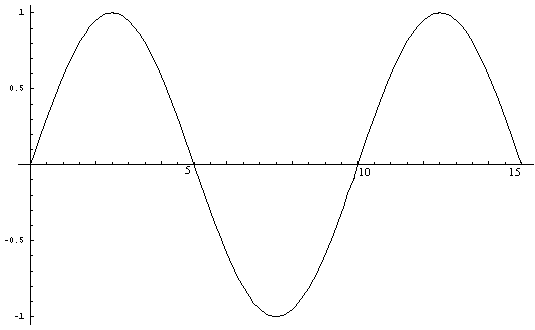# Formulae for DeterminingPrimality or Compositeness

by Charles Dean Pruitt
(with trigonometric prompting by Katya A.)
ABSTRACT:
The following is a method to produce formulas of various complexities
with real coeffecients whose itereation indicate whether a given integer
is prime or composite.

METHOD:
From theory and by inspection, composite numbers with similar
factors appear on the number line in a periodic fashion.
Conversely prime numbers appear on the number line irregularly as "not composites".
Therefore it is possible to find a periodic function such as a sine curve or cycloid with
a particular periodicity which will cross the x-axis (on a two-dimensional Cartesian
coordinate system) at each integer whose factors correspond to that periodicity.
For purposes of this argument the sine curve is used because it is the most familiar.
For example: the sine curve (Sin xPi/2) has an amplitude of one and passes through
the points on the x-axis (0,0), (2,0), (4,0), (6,0) to infinity, i.e. multiples of two.Figure 1. (Sin xPi/2)

Likewise the sine curve (Sin xPi/3) has an amplitude of one and passes through
the points on the x-axis (0,0), (3,0), (6,0), (9,0) to infinity, i.e. multiples of three.Figure 2. (Sin xPi/3)

In the same way, the sine curve (Sin xPi/5) has an amplitude of one and passes through
the points on the x-axis (0,0), (5,0), (10,0), (15,0) to infinity, i.e. multiples of five.Figure 3. (Sin xPi/5)

From each of the above three figures it can be seen that the sine curve passes through
all points on the x-axis which have its respective prime (and periodicity) as a factor.
When Figure 1 is multiplied by Figure 2, Figure 4 results.
This is the graph of the curve (Sin xPi/2 * Sin xPi/3).
It is possible to see that the zero points where the curve crosses the x-axis
correspond to the integers which have 2 or 3 or both as a factor.
Where the curve does not intersect the x-axis at the integer values
are integers relatively prime to two or three.
This is the case up until 'x' equals 25 or five squared.
This is consistent with standard number theory concerning factorability.
Therefore for the equation
Sin xPi/2 * Sin xPi/3 = 0,
the points which satisfy the equation for 'x' are
(2,0), (3,0), (4,0), (6,0), (8,0), (9,0), (10,0), (12,0), (14,0), (15,0),
(16,0), (18,0), (20,0), (21,0), (22,0), (24,0).
By inspection (see Figure 4), it can be seen that there is no intersection with
the curve at the points on the x-axis
(5,0), (7,0), (11,0), (13,0), (17,0), (19,0), (23,0).
This is confirmed when these values for 'x' are substituted into
the equation and non-zero answers result.
In effect these are all the primes less than five squared except two and three.
By the above method is it possible to generate equations which when solved for
x =0 indicate that all integers left over are primes.Figure 4. (Sin xPi/2 * Sin xPi/3)

In order to find the equation which will provide the curve which
will cross the x-axis for all non-primes less than seven squared,
it is necessary to multiply (Sin xPi/2 * Sin xPi/3 * Sin xPi/5).Figure 5. (Sin xPi/2 * Sin xPi/3 * Sin xPi/5)

Although it is not as clear as on the other graphs,
it is possible to recognize the prime points on the x-axis.
Therefore when solving for
Sin xPi/2 * Sin xPi/3 * Sin xPi/5 = 0,
the solutions for 'x' (when x<49) comprise all the composite numbers
less than 7 squared as well as 2, 3, and 5.
Therefore all the remaining integers are prime.
With 2, 3, and 5 this constitutes all the primes less than 49.
It is possible to check this empirically by substitution.
For exceedingly large samples it is not useful to view a whole graph
because the fine details are not readily clear.
However it is possible to calculate for a large range
by multiplying all the consecutive sine curves together.
In the following example the first 27 sine curves (Sin xPi/2) through (Sin xPi/103)
were multiplied together to yield a graph for the resultant curve
of which 100 units are shown below.
That curve is stated as:
Sin xPi/2 * Sin xPi/3 * Sin xPi/5 * Sin xPi/7 * Sin xPi/11 * Sin xPi/13 * Sin xPi/17 *
Sin xPi/19 * Sin xPi/23 * Sin xPi/29 * Sin xPi/31 * Sin xPi/37 * Sin xPi/41 * Sin xPi/43 *
Sin xPi/47 * Sin xPi/53 * Sin xPi/59 * Sin xPi/61 * Sin xPi/67 * Sin xPi/71 * Sin xPi/73 *
Sin xPi/79 * Sin xPi/83 * Sin xPi/89 *Sin xPi/97 *Sin xPi/101 * Sin xPi/103
(Equation A.)
Solving the resultant equation for zero yields all the
composite numbers up to 103 squared (10609) and
consequently all of the primes less than 10609.Figure 6. (Sin xPi/2* Sin xPi/3 * ... * Sin xPi/101 * Sin xPi/103)

For purposes of illustration a small segment of the graph
is blown up (figure 7) to shown the fine detail and confirm
visually that indeed the resulting curve crosses the x-axis
at all the composite numbers and not at any of the primes.
However for all values of 'x' which satisfy the equation,
results can only be derived by solving for zero.Figure 7.

It appears from this segment of the graph that 10007 and 10009
are prime (and constitute a prime pair). This is indeed the case.
The curve between 10004 and 10006 has an amplitude of less than one ten-billionth.
With further magnification (see Figure 8) details as fine one part in a trillion
are resolved and indicate 10005 is composite.
(Of course there are easier methods to determine
compositeness of numbers ending in 5.)Figure 8. Detail 10003-10006

Therefore, the method to demonstrate primality or compositeness is
to construct an appropriate equation of periodic curves multiplied together
as above so that the final curve multiplied with all the initial curves
is Sin xPi/A where 'A' is the first prime greater
than the square root of the number being tested.
Sin xPi/2*Sin xPi/3*Sin xPi/5*...*Sin xPi/A
Substituting the number to be tested for 'x' yield zero if the number
is a composite and a real non-zero value if the number is prime.

PROOF

Each periodic function as defined above intersects
the x-axis at points that correspond to all
the integers divisible by that function when y=0.
Sin xPi/2=0 represents all integers divisible by 2.
Sin xPi3=0 represents all integers divisible by 3.
Sin xPi/5=0 represents all integers divisible by 5.
When two functions are multiplied together,
they will show up all the zeroes because 0 times anything is 0 .
Sin xPi/2 * Sin xPi/3=0 represents all integers divisible by 2 and/or 3.
Sin xPi/3 * Sin xPi/5=0 represents all integers divisible by 3 and/or 5.
Sin xPi/5 * Sin xPi/7=0 represents all integers divisible by 5 and/or 7.
There is not duplication because 0 times 0 is 0
and hence there would be only one point indicated instead of two.
Likewise the same argument applies when many sine curves are multiplied together.
When all sine curves (each with periodicity corresponding to a prime and
all such curves up to and including that whose periodicity is
less than or equal to the square root of the largest number
in the sample space being tested) are multiplied together,
the resultant equation gives each composite number one time
when the equation is solved for zero.
Discarding these from the set of integers leaves the primes.
In practice this has become a formulaic Sieve of Erastathenes.

COMMENTARY

Are these true formulae for testing primality? Yes.
Are they useful formulae? In their current form they are trivial.
They are computationally intensive, but the idea of multiplying
periodic equations together and solving for zero has utility value.
Suppose periodic equations could be constructed that were inherently simple
and when multiplied together were likewise simple.
The restraint of computational intensity would be diminished
with a clever enough set of periodic equations.
If non-trigonometric periodic equations could be found,
it might produce a polynomial which could be solved for
and produce integer roots all of which are composite.
From this would come all the primes via solution of a polynomial
and subsequent cataloging those integers which don't solve the equation.
By way of example, Equation A can test
all numbers less than 10609 for primality or compositeness.
Substitute the number to be tested for 'x'.
If the answer is zero the number is composite.
If the answer is not zero the number is prime.
As mentioned before, the possibility to find simpler or
more clever periodic equations would reduce the computational intensity.
Another possibility is to choose periodic formulas where the
results can be simplified using trigonometric identities.
Whether the computation intensiveness can be reduced
low enough (i.e. linear instead of multiplicative or exponential)
is an open question.